# What is Schottky diode and its applications

A Schottky diode is also known as surface barrier diode , hot carrier diode or hot electron diode. It has a lower power drop across it than a normal p-n junction diode which is due to the diode design. It is a unipolar diode as in only one of the charge carriers are involved in the working. Applications range from radio frequency applications to power applications.

## What is a Schottky Diode?

A Schottky diode is a semiconducting device that has very fast switching action. Diodes are normally used as switches where they turn on and off depending on the voltage drop (0.6 to 1.7 v) across it. Having a low voltage drop (0.6 to 1.7 v ) helps with faster switching in Schottky diodes. This switching action improves the efficiency of the system.

An ordinary diode is a sandwich of a p and a n type semiconductor layers acting as the cathode and anode being unidirectional. As current flows through the device, a potential barrier is developed at the junction of the layers which is the voltage drop.

But a Schottky diode is a semiconductor- metal sandwich hence the potential barrier is not as prominent. The n type semiconductor acts as the cathode and the metal as the anode.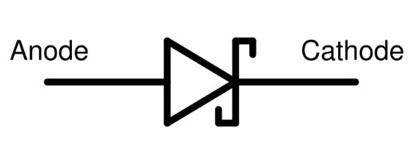### What Is Schottky barrier

Thought the potential barrier is less, at the junction there is a formation of a depletion layer with electrons at the metal side which attracts the holes in the n type material pushing the electrons to the other side. Until an equilibrium with the energy bands of the metal and semiconductor is reached. The barrier is formed at the semiconductor region because there are already free electrons in the metals. This barrier formed is called the Schottky barrier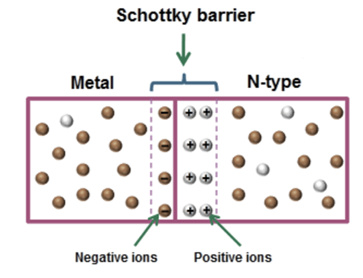## Schottky Diode Construction

Ideally from the construction of the Schottky diode, there are two metal semiconductor junctions formed, i.e one between the metal and lightly doped n type semiconductor layer and another between heavily doped semiconductor and the metal layer.

A junction between the heavily doped semiconductor region and the metal is called ohmic contact . In this junction the barrier height (ie the activation energy required) is less hence the electrons can easily move around in the ohmic contact i.e. the electrons become directional with linear V-I characteristics. This ohmic contact is between the semiconductor and the external contact wire.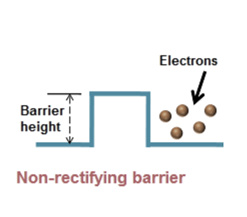A junction between the lightly doped semiconductor and the metal is called a rectifying junction , where the barrier potential is high. Hence electrons need external additional push to flow through, The V-I characteristics of this junction is nonlinear.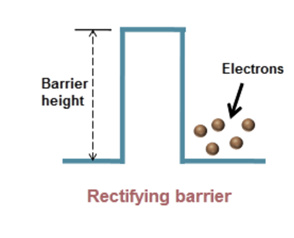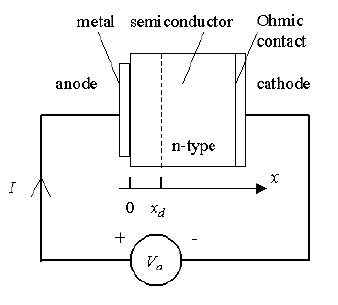## Structure of Schottky Diode

Since these are unipolar devices that depends on the electron flow in them, the parameters of a Schottky diode is determined by the doping concentration of the semiconductor layer.

Ideally Platinum, Tungsten, Gold etc are used as the metal in combination with the n type semiconductor.

At the rectifying junction, near the barrier the current flow is unidirectional and conducting only after the the external voltage is greater than the voltage drop, hence it can be used in applications where clamping and rectification is needed at lower voltage supply.

### Current Components in Schottky Diode

The current flow in Schottky barrier diode due to the flow of majority carriers i.e. the electrons is given by

IT=IDiffusion + ITunneling + IThermionic emission

Where

*  IDiffusion -  diffusion current

Diffusion current is based on the concentration gradient of the carriers over the length of the semiconductor and the diffusion current density.

diffusion current density is given as  Jn=Dn*q*dn/dx  for electrons,

where

Dn - diffusion constant of electrons,

q is electronic charge = 1.6*1019coulombs,

dn/dx is a concentration gradient for electrons.

* ITunneling -  tunnelling current.

In quantum mechanics tunnelling is a phenomenon where the electrons can tunnel trough the barrier when the excitation energy or activation energy to cross the potential Is very high .This follows that the electrons could tunnels through at the barrier where the potential begins but the probability decreases as the width of this layer of build In potential ( called the depletion layer) increases

* IThermionic emission - a current due to thermionic emission current.

Every element has a conduction band and valence band. while these bands intersect for metals, they are distinct and wide apart in semiconductors, at the metal – semiconductor junction they are in the intermediate level. Temperature can cause more electrons to be developed from the break down of the electron hole pair in the semiconductor. As temperature increases more electrons are produced and hence more current is produced.

## Forward biased schottky diode

When the positive terminal of a DC supply is connected to the anode (metal) and the negative terminal to the cathode (semiconductor),the setup is in forward bias.

When voltage is applied, the electrons in the n type region move towards the barrier and when the voltage is greater than the barrier potential, the electrons flow to the metal, also more electrons are produced in the n- type semiconductor.

## Reverse bias schottky diode

Connecting the positive terminal of the DC supply to the semiconductor and the negative terminal to the metal is reverse biasing.

When a reverse bias voltage is applied to the Schottky diode, due to the positive potential at the n-type semiconductor, the electrons migrate to the ohmic junction thus the depletion width increases. consequently, the electric current stops flowing. So, when the diffusion current and tunnelling current does not flow due to the width of the junction, thermally excited electrons can move through. This is because as the voltage is increased the temperature in the materials also increases due to the resistance.

The V-I characteristics of a Schottky barrier diode are below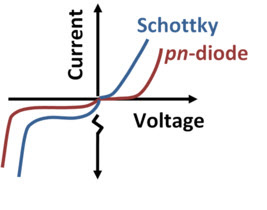• The forward voltage drop in the Schottky diode is less than the p-n junction diode • The current curve is steeper too because of the high concentration of the n type carriers( electrons) • As the concentration of electrons increases voltage drop will increase.

## Applications Of Schottky Diode

1. voltage clamping applications When transistors are in the saturation mode, using it as a switch is difficult due to its high switch off time which is because of the large charge accumulated in the base. For fast switching action, this accumulated charge should be depleted which is done using baker clamp circuits that use the fast recovery time and switching capabilities of the Schottky diode. Thus, preventing transistor saturation.
2. By pass diodes : In solar cell applications when one cell stops working due to shade, it will act as a power absorber instead of a power emitter and will cause huge current to flow through it which will damage the cell. To prevent this, diode are placed parallel to the cell and using a low voltage switching Schottky diode will help in burn out prevention. In the same way it can be used in offgrid systems to prevent current from battery to leak out when the cells do not work at night. They are also used as free wheeling diodes.
3. Rectifers for power supply.
4. Sample and hold circuit They help in faster transition from sample to hold than regular diodes, also due to faster switching with electrons alone as carriers , more accurate sampling can be done

## Advantages Of Schottky Diode

Low turn on voltage: The turn on voltage for the diode is between 0.2 and 0.3 volts. For a silicon diode it is against 0.6 to 0.7 volts from a standard silicon diode.

Fast recovery time: A fast recovery time means a small amount of stored charge that can be used for high speed switching applications.

Low junction capacitance: The barrier, which can store energy acting as capacitor occupies a very small area, hence the capacitance levels are very small. The time constant is a function of resistance and capacitance. So it makes the system faster.

High Efficiency: Because of the low voltage needed, less energy is used and hat dissipation and subsequent loss of energy is avoided.